CBSE Class 7CBSE
Share

NCERT solutions for Class 7 Mathematics chapter 14 - Symmetry

Mathematics for Class 7

NCERT Mathematics Class 7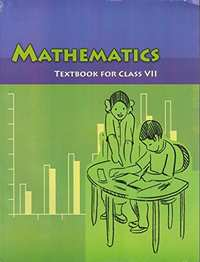Chapter 14: Symmetry

Ex. 14.10Ex. 14.20Ex. 14.30

Chapter 14: Symmetry Exercise 14.10 solutions [Pages 268 - 270]

Ex. 14.10 | Q 1.01 | Page 268

Copy the figure with punched holes and find the axes of symmetry for the followingEx. 14.10 | Q 1.02 | Page 268

Copy the figure with punched holes and find the axes of symmetry for the followingEx. 14.10 | Q 1.03 | Page 268

Copy the figure with punched holes and find the axes of symmetry for the following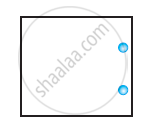Ex. 14.10 | Q 1.04 | Page 268

Copy the figure with punched holes and find the axes of symmetry for the following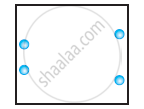Ex. 14.10 | Q 1.05 | Page 268

Copy the figure with punched holes and find the axes of symmetry for the following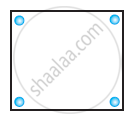Ex. 14.10 | Q 1.06 | Page 268

Copy the figure with punched holes and find the axes of symmetry for the following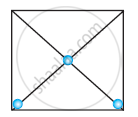Ex. 14.10 | Q 1.07 | Page 268

Copy the figure with punched holes and find the axes of symmetry for the following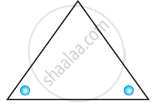Ex. 14.10 | Q 1.08 | Page 268

Copy the figure with punched holes and find the axes of symmetry for the following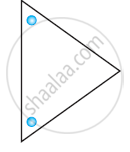Ex. 14.10 | Q 1.09 | Page 269

Copy the figure with punched holes and find the axes of symmetry for the following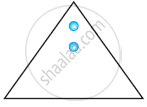Ex. 14.10 | Q 1.1 | Page 268

Copy the figure with punched holes and find the axes of symmetry for the following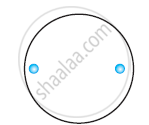Ex. 14.10 | Q 1.11 | Page 268

Copy the figure with punched holes and find the axes of symmetry for the followingEx. 14.10 | Q 1.12 | Page 268

Copy the figure with punched holes and find the axes of symmetry for the following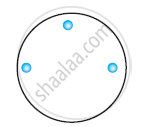Ex. 14.10 | Q 2.05 | Page 269

Given the line(s) of symmetry, find the other hole(s):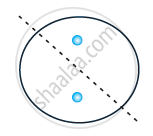Ex. 14.10 | Q 2.1 | Page 269

Given the line(s) of symmetry, find the other hole(s):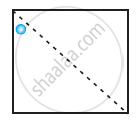Ex. 14.10 | Q 2.2 | Page 269

Given the line(s) of symmetry, find the other hole(s):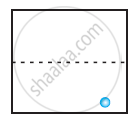Ex. 14.10 | Q 2.3 | Page 269

Given the line(s) of symmetry, find the other hole(s):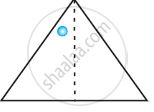Ex. 14.10 | Q 2.4 | Page 268

Given the line(s) of symmetry, find the other hole(s):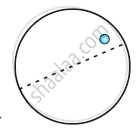Ex. 14.10 | Q 3.1 | Page 269

In the given figure, the mirror line (i.e., the line of symmetry) is given as a dotted line. Complete given figure performing reflection in the dotted (mirror) line. (You might perhaps place a mirror along the dotted line and look into the mirror for the image). Are you able to recall the name of the figure you complete?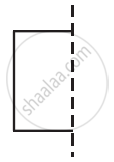Ex. 14.10 | Q 3.2 | Page 269

In the given figure, the mirror line (i.e., the line of symmetry) is given as a dotted line. Complete given figure performing reflection in the dotted (mirror) line. (You might perhaps place a mirror along the dotted line and look into the mirror for the image). Are you able to recall the name of the figure you complete?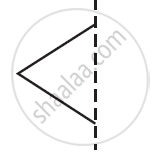Ex. 14.10 | Q 3.3 | Page 269

In the given figure, the mirror line (i.e., the line of symmetry) is given as a dotted line. Complete given figure performing reflection in the dotted (mirror) line. (You might perhaps place a mirror along the dotted line and look into the mirror for the image). Are you able to recall the name of the figure you complete?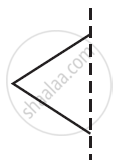Ex. 14.10 | Q 3.4 | Page 269

In the given figure, the mirror line (i.e., the line of symmetry) is given as a dotted line. Complete given figure performing reflection in the dotted (mirror) line. (You might perhaps place a mirror along the dotted line and look into the mirror for the image). Are you able to recall the name of the figure you complete?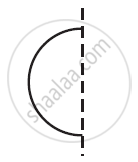Ex. 14.10 | Q 3.5 | Page 269

In the given figure, the mirror line (i.e., the line of symmetry) is given as a dotted line. Complete given figure performing reflection in the dotted (mirror) line. (You might perhaps place a mirror along the dotted line and look into the mirror for the image). Are you able to recall the name of the figure you complete?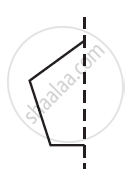Ex. 14.10 | Q 3.6 | Page 269

In the given figure, the mirror line (i.e., the line of symmetry) is given as a dotted line. Complete given figure performing reflection in the dotted (mirror) line. (You might perhaps place a mirror along the dotted line and look into the mirror for the image). Are you able to recall the name of the figure you complete?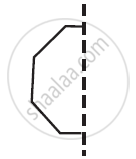Ex. 14.10 | Q 4.1 | Page 269

The following figures have more than one line of symmetry. Such figures are said to have multiple lines of symmetry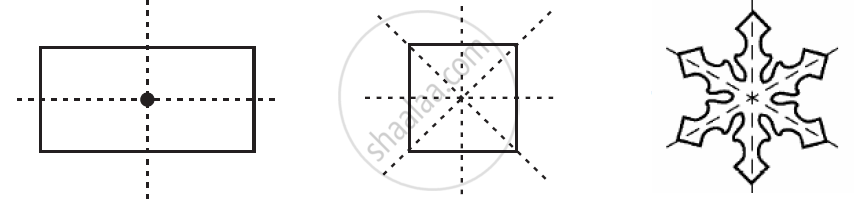Identify multiple lines of symmetry, if any, in the given figure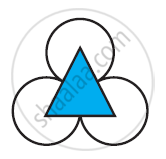Ex. 14.10 | Q 4.2 | Page 269

The following figures have more than one line of symmetry. Such figures are said to have multiple lines of symmetryIdentify multiple lines of symmetry, if any, in the given figure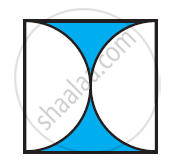Ex. 14.10 | Q 4.3 | Page 269

The following figures have more than one line of symmetry. Such figures are said to have multiple lines of symmetryIdentify multiple lines of symmetry, if any, in the given figure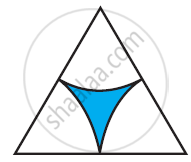Ex. 14.10 | Q 4.4 | Page 269

The following figures have more than one line of symmetry. Such figures are said to have multiple lines of symmetryIdentify multiple lines of symmetry, if any, in the given figureEx. 14.10 | Q 4.5 | Page 270

The following figures have more than one line of symmetry. Such figures are said to have multiple lines of symmetryIdentify multiple lines of symmetry, if any, in the given figure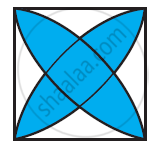Ex. 14.10 | Q 4.6 | Page 270

The following figures have more than one line of symmetry. Such figures are said to have multiple lines of symmetryIdentify multiple lines of symmetry, if any, in the given figure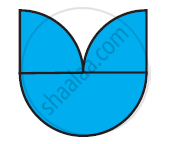Ex. 14.10 | Q 4.7 | Page 270

The following figures have more than one line of symmetry. Such figures are said to have multiple lines of symmetryIdentify multiple lines of symmetry, if any, in the given figure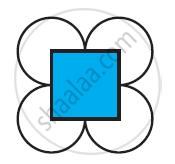Ex. 14.10 | Q 4.8 | Page 270

The following figures have more than one line of symmetry. Such figures are said to have multiple lines of symmetryIdentify multiple lines of symmetry, if any, in the given figure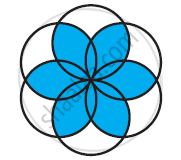Ex. 14.10 | Q 5 | Page 270

Copy the figure given hereTake any one diagonal as a line of symmetry and shade a few more squares to make the figure symmetric about a diagonal. Is there more than one way to do that? Will the figure be symmetric about both the diagonals?

Ex. 14.10 | Q 6.1 | Page 270

Copy the diagram and complete the given shape to be symmetric about the mirror line(s):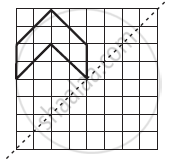Ex. 14.10 | Q 6.2 | Page 270

Copy the diagram and complete the given shape to be symmetric about the mirror line(s):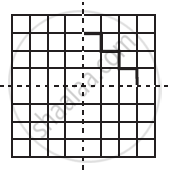Ex. 14.10 | Q 6.3 | Page 270

Copy the diagram and complete the given shape to be symmetric about the mirror line(s):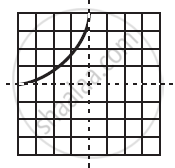Ex. 14.10 | Q 7.01 | Page 270

State the number of lines of symmetry for An equilateral triangle figure

Ex. 14.10 | Q 7.02 | Page 270

State the number of lines of symmetry for An isosceles triangle figure

Ex. 14.10 | Q 7.03 | Page 270

State the number of lines of symmetry for A scalene triangle figure

Ex. 14.10 | Q 7.04 | Page 270

State the number of lines of symmetry for A square figure

Ex. 14.10 | Q 7.05 | Page 270

State the number of lines of symmetry for A rectangle figure

Ex. 14.10 | Q 7.06 | Page 270

State the number of lines of symmetry for A rhombus figure

Ex. 14.10 | Q 7.07 | Page 270

State the number of lines of symmetry for A parallelogram figure

Ex. 14.10 | Q 7.08 | Page 270

State the number of lines of symmetry for A quadrilateral figure

Ex. 14.10 | Q 7.09 | Page 270

State the number of lines of symmetry for A regular hexagon figure

Ex. 14.10 | Q 7.1 | Page 270

State the number of lines of symmetry for A circle figure

Ex. 14.10 | Q 8.1 | Page 270

What letters of the English alphabet have reflectional symmetry (i.e., symmetry related to mirror reflection) about a vertical mirror

Ex. 14.10 | Q 8.2 | Page 270

What letters of the English alphabet have reflectional symmetry (i.e., symmetry related to mirror reflection) about a horizontal mirror

Ex. 14.10 | Q 8.3 | Page 270

What letters of the English alphabet have reflectional symmetry (i.e., symmetry related to mirror reflection) about both horizontal and vertical mirrors

Ex. 14.10 | Q 9 | Page 270

Give three examples of shapes with no line of symmetry.

Ex. 14.10 | Q 10.1 | Page 270

What other name can you give to the line of symmetry of an isosceles triangle?

Ex. 14.10 | Q 10.2 | Page 270

What other name can you give to the line of symmetry of a circle?

Chapter 14: Symmetry Exercise 14.20 solutions [Page 274]

Ex. 14.20 | Q 1.1 | Page 274

The following figure have rotational symmetry of order more than 1: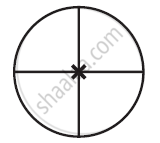Ex. 14.20 | Q 1.2 | Page 274

The following figure have rotational symmetry of order more than 1: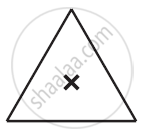Ex. 14.20 | Q 1.3 | Page 274

The following figure have rotational symmetry of order more than 1: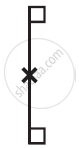Ex. 14.20 | Q 1.4 | Page 274

The following figure have rotational symmetry of order more than 1: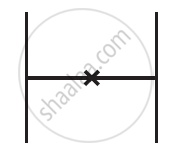Ex. 14.20 | Q 1.5 | Page 274

The following figure have rotational symmetry of order more than 1: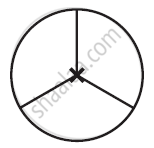Ex. 14.20 | Q 1.6 | Page 274

The following figure have rotational symmetry of order more than 1: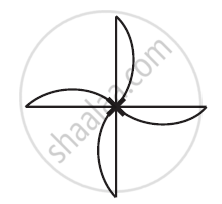Ex. 14.20 | Q 2.1 | Page 274

Give the order of rotational symmetry for given figure: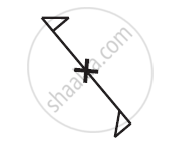Ex. 14.20 | Q 2.2 | Page 274

Give the order of rotational symmetry for given figure:Ex. 14.20 | Q 2.3 | Page 274

Give the order of rotational symmetry for given figure: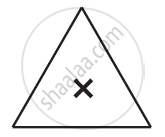Ex. 14.20 | Q 2.4 | Page 274

Give the order of rotational symmetry for each figure: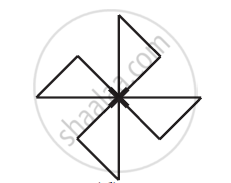Ex. 14.20 | Q 2.5 | Page 274

Give the order of rotational symmetry for given figure: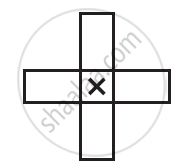Ex. 14.20 | Q 2.6 | Page 274

Give the order of rotational symmetry for given figure: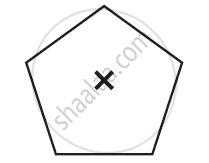Ex. 14.20 | Q 2.7 | Page 274

Give the order of rotational symmetry for given figure:Ex. 14.20 | Q 2.8 | Page 274

Give the order of rotational symmetry for given figure: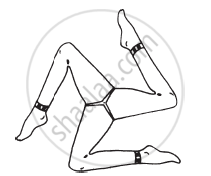Chapter 14: Symmetry Exercise 14.30 solutions [Page 275]

Ex. 14.30 | Q 1 | Page 275

Name any two figures that have both line symmetry and rotational symmetry.

Ex. 14.30 | Q 2.1 | Page 275

Draw, wherever possible, a rough sketch of a triangle with both line and rotational symmetries of order more than 1.

Ex. 14.30 | Q 2.2 | Page 275

Draw, wherever possible, a rough sketch of a triangle with only line symmetry and no rotational symmetry of order more than 1.

Ex. 14.30 | Q 2.2 | Page 275

Draw, wherever possible, a rough sketch of a quadrilateral with a rotational symmetry of order more than 1 but not a line symmetry.

Ex. 14.30 | Q 2.4 | Page 275

Draw, wherever possible, a rough sketch of a quadrilateral with line symmetry but not a rotational symmetry of order more than 1

Ex. 14.30 | Q 3 | Page 275

If a figure has two or more lines of symmetry, should it have rotational symmetry of order more than 1?

Ex. 14.30 | Q 4 | Page 275

Fill in the blanks:

 Shape Centre of Rotation Order of Rotation Angle of Rotation Square - - - Rectangle - - - Rhombus - - - Equilateral Triangle - - - Regular Hexagon - - - Circle - - - Semi-circle - - -

Chapter 14: Symmetry

Ex. 14.10Ex. 14.20Ex. 14.30

NCERT Mathematics Class 7NCERT solutions for Class 7 Mathematics chapter 14 - Symmetry

NCERT solutions for Class 7 Maths chapter 14 (Symmetry) include all questions with solution and detail explanation. This will clear students doubts about any question and improve application skills while preparing for board exams. The detailed, step-by-step solutions will help you understand the concepts better and clear your confusions, if any. Shaalaa.com has the CBSE Mathematics for Class 7 solutions in a manner that help students grasp basic concepts better and faster.

Further, we at Shaalaa.com are providing such solutions so that students can prepare for written exams. NCERT textbook solutions can be a core help for self-study and acts as a perfect self-help guidance for students.

Concepts covered in Class 7 Mathematics chapter 14 Symmetry are Introduction of Symmetry, Lines of Symmetry for Regular Polygons, Concept of Rotational Symmetry, Line Symmetry and Rotational Symmetry.

Using NCERT Class 7 solutions Symmetry exercise by students are an easy way to prepare for the exams, as they involve solutions arranged chapter-wise also page wise. The questions involved in NCERT Solutions are important questions that can be asked in the final exam. Maximum students of CBSE Class 7 prefer NCERT Textbook Solutions to score more in exam.

Get the free view of chapter 14 Symmetry Class 7 extra questions for Maths and can use Shaalaa.com to keep it handy for your exam preparation

S